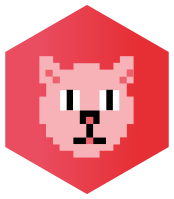Online
Classroom

Scala Cats and Cats-Effect 2.x

Part of our scala Courses

2 daysCourse Overview

With this course we will be covering a range of topics that will give you a solid grounding in Scala Cats and Cats-Effect 2. If you are looking for a new version of this course that uses Cats Effect 3, then checkout the SCALA-CATS course. In this course, you will not only will you receive an introduction to Category Theory, Cats, Cats Effects, but we will also go through Tagless Final Encoding to give you a well-rounded overview. This course has been designed to give you as comprehensive an introduction as possible while still leaving time and space for questions and discussion.

Course Prerequisites

Students should be familiar with the Scala programming language (preferable version 3). You should in particular be familiar with aspects such as type-classes and context bounds. It is a great course for student preparing to use Scala libraries such as fs2, http4s, ZIO, akka etc

Outline

Scala Recap (if needed)

• Type constructors
• Higher-kinded types
• Type classes in Scala
• Context bounds

Introduction to Cats

• Understand the scope and intent of Cats
• Explain the structure of the library
• List cats modules
• Various import approaches
• List and discuss related projects
• Using a cats Type class
• Discuss ID Operations
• List various small enhancements to Scala (e.g, for Either/Option)
• Introduce Monoids and Semigroups

Overview of Category Theory

• Introduce Category Theory for Developers
• What is a category
• Understand objects and morphisms
• Define composition and associativity
• Introduce the identity arrow
• Introduce the term Hom-Set
• Discuss Example Categories (0 , 1, 2, Monoid, Set)
• Understand the category of types and functions
• Discuss and implement Kleisli as an example
• Initial and Terminal Objects
• Discuss the concept of Universal Construction
• Discuss and implement Product Types
• Introduce natural transformations

Cats Functors, Applicatives, Traverse and Monads

Functors

• Introduce Functors in terms of Categories
• Use Endofunctors in Programming
• Introduce the functor laws
• Introduce the Cats Functor Type class
• Using derived methods (lift, as, …)
• Introduce Natural Transformations
• Understand FunctionK
• Combing Functors using the Nested type
• Introduce Bifunctors

Applicatives and Traverse

• Introduce the Applicative functor
• compose independent effects
• Using product/tuple functions
• Using mapN functions
• Replicating Applicatives
• Composing Applicatives
• Introduce Traverse
• Using Traverse sequence

• Recap Kleisli
• composing dependent effects using Monads

Cats Core Data Types

Validated

• Using Validated to accumulate errors
• Understand the role of sequence
• Using the Chain and NonEmptyChain

Parallel

• Understand the need to Parallel
• Recap FunctionK and Natural Transformations
• Using parallel operations on Monads (e.g, parProduct, parSequence etc)

Eval

• Using Eval to control synchronous evaluation
• Understand the concept of trampolining
• Understand the concepts of now, later, always
• Using defer to lift a value into 'Eval'

• Understand the role of Monad Transformers
• Use transformers to stack effects
• List bundled transformers (OptionT, EitherT, ..)
• Caveats and alternatives

• Use the Reader for implicit contextual objects (e.g, configuration)
• Using the Reader for dependency injection (with effect tracking)
• Using ReaderT to stack other effects
• Using the writer for audit trails

Raising and Handling Errors

• Introduce the ApplicativeError and MonadError
• Raising errors
• Handling errors
• Transforming errors (e.g, to Either)
• Using an error-tap

Dealing with Side Effects (Cats Effect)

• What are effects?
• Challenges with effects and Functional Programming
• Side effects and Referential Transparency
• Introduce Cats Effect
• Understand the relation with Cats

• Introduce the IO Monad reference implementation
• Trampolining and IO
• Understand the different execution styles (pure, lazy, async)
• Introduce the IOApp

The Effect Type Classes

• Recap Sync, Async and Concurrent
• List and introduce the other type classes (e.g, Bracker, Effect, …)
• Writing polymorphic code using context bounds
• Discuss logging (log4cats)

Resource Management

• Explore the Bracket type
• Understand nested brackets
• Using the Resource type

ContextShift

• Understand the role of ContextShift
• Understand the role of thread shifting on cancellation
• Understand Fibers
• Using Blocker for I/O bound code

Concurrency

• Recap of concurrency theory
• Understand the problems with JVM Threads and Concurrency
• Discuss best practices for application thread-pools
• Introduce and use Clock and Timer
• How to use fork, join and cancel
• Introduce the low-level Concurrence Structures (Deferred, MVar2, Ref, Semaphore)
• How to write Cancelable jobs
• Introduce high-level apis
• Use the cats Parallel type class for parallel execution
• Build race conditions
• Integrate with legacy code (Futures)

Tagless Final

• Understand the theory behind TF
• Discuss Initial vs Final encoding
• Appreciate Tagged vs Tagless
• Encode your algebra using TF
• Discuss laws
• Using TF with Cats/ Cats Effect
• Discuss various Encoding styles (functions, modules, traits etc)

Public Events

Currently we have no public courses planned for Scala Cats and Cats-Effect 2.x

Private Events

Do you have a team that needs a Scala Cats and Cats-Effect 2.x course?